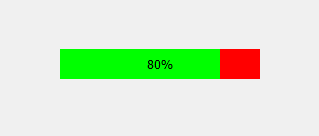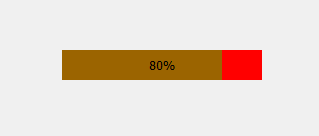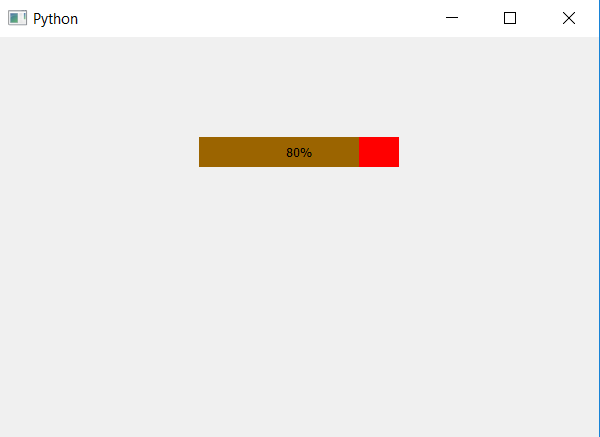GeeksforGeeks App
Open AppBrowser
Continue

# PyQt5 – Translucent Bar of Progress Bar

In this article we will see how to make the bar translucent i.e in between opaque and transparent. The progress bar have two components one is the background which is visible when bar is not at 100% other is the bar which tells the progress, when we make bar translucent the background will be visible.

In order to do this we have to change the alpha level i.e transparency level of bar, below is normal progress bar vs translucent progress bar, background color is set to red and bar color is set to green.In order to change the alpha level we have to change the CSS style sheet, below is the style sheet code for bar.

```QProgressBar::chunk
{
background : rgba(0, 255, 0, 100);
}
```

This sheet is used which `setStyleSheet` method, below is the implementation

 `# importing libraries``from` `PyQt5.QtWidgets ``import` `*` `from` `PyQt5 ``import` `QtCore, QtGui``from` `PyQt5.QtGui ``import` `*` `from` `PyQt5.QtCore ``import` `*` `import` `sys`` ` ` ` `class` `Window(QMainWindow):`` ` `    ``def` `__init__(``self``):``        ``super``().__init__()`` ` `        ``# setting title``        ``self``.setWindowTitle(``"Python "``)`` ` `        ``# setting background color to window``        ``# self.setStyleSheet("background-color : yellow")`` ` `        ``# setting geometry``        ``self``.setGeometry(``100``, ``100``, ``600``, ``400``)`` ` `        ``# calling method``        ``self``.UiComponents()`` ` `        ``# showing all the widgets``        ``self``.show()`` ` `    ``# method for widgets``    ``def` `UiComponents(``self``):``        ``# creating progress bar``        ``bar ``=` `QProgressBar(``self``)`` ` `        ``# setting geometry to progress bar``        ``bar.setGeometry(``200``, ``100``, ``200``, ``30``)`` ` `        ``# setting the value``        ``bar.setValue(``80``)`` ` `        ``# setting alignment to center``        ``bar.setAlignment(Qt.AlignCenter)`` ` `        ``# setting background to color ``        ``# and bar color with alpha factor``        ``bar.setStyleSheet(``"QProgressBar"``                          ``"{"``                            ``"background-color : rgba(255, 0, 0, 255);"``                            ``"border : 1px"``                          ``"}"`` ` `                          ``"QProgressBar::chunk"``                          ``"{"``                            ``"background : rgba(0, 255, 0, 100);"``                          ``"}"``                          ``)`` ` ` ` `App ``=` `QApplication(sys.argv)`` ` `# create the instance of our Window``window ``=` `Window()`` ` `# start the app``sys.exit(App.``exec``())`

Output :My Personal Notes arrow_drop_up